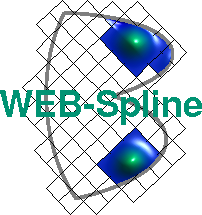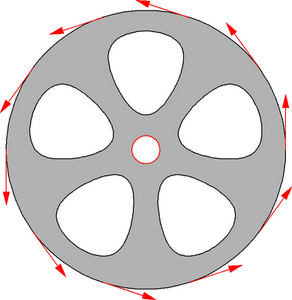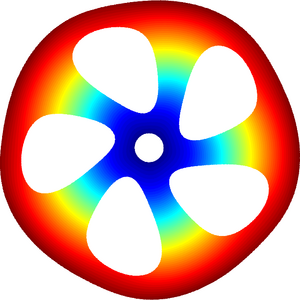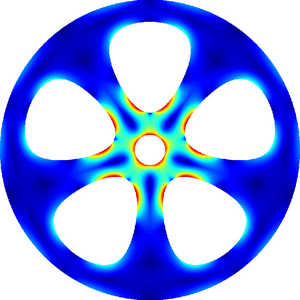# Linear Elasticity: Plane Stress

Wheel with uniform tangential load on outer boundary, fixed at the hub, inner holes unloaded.The numerical solution is computed via the displacement model. Moreover, it is assumed that the z-components of the forces vanish (plane stress model). WEB-splines of degree 3 on a grid of 156x156 cells have been used. The fixation was modeled by w(x,y)=x2+y2-r2, where r denotes the hub radius.

The following figures visualize the magnified displacement (left) and the maximum principal stress (right).### Literature

K. Höllig: Finite Element Methods with B-Splines. Frontiers in Applied Mathematics 26, SIAM, 2003.

See Publications for a complete list of WEB-publications.

Author: Joachim Wipper ; Last modification: 2006/08/31 09:36:36 UTC.

 [Home] [The WEB-Method] [Examples] [Publications] [Contact]